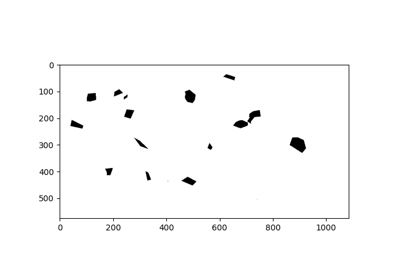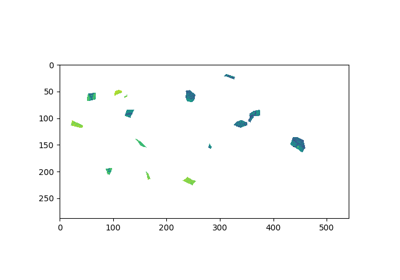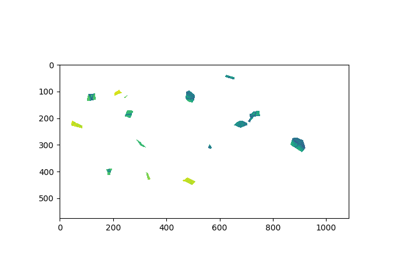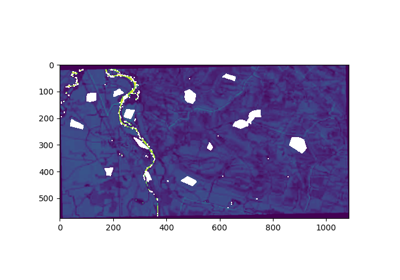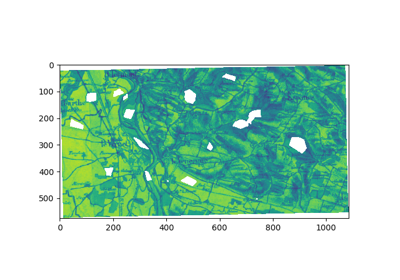`museotoolbox.processing.``image_mask_from_vector`(in_vector, in_image, out_image, invert=False, gdt=1)[source]

Create a image mask where polygons/points are the pixels to keep.

Parameters
• in_vector (str.) – Path of the vector file to rasterize.

• in_image (str.) – Path of the raster file where the vector file will be rasterize.

• out_image (str.) – Path of the file (.tif) to create.

• invert (bool, optional (default=False)) – invert=True make polygons/points with 0 values in out_image.

• gdt (int, optional (default=gdal.GDT_Byte)) – The gdal datatype of the rasterized vector.

## Examples using `museotoolbox.processing.image_mask_from_vector`¶